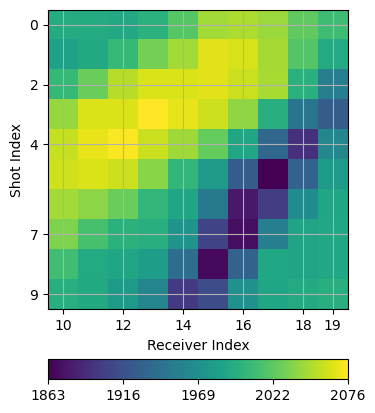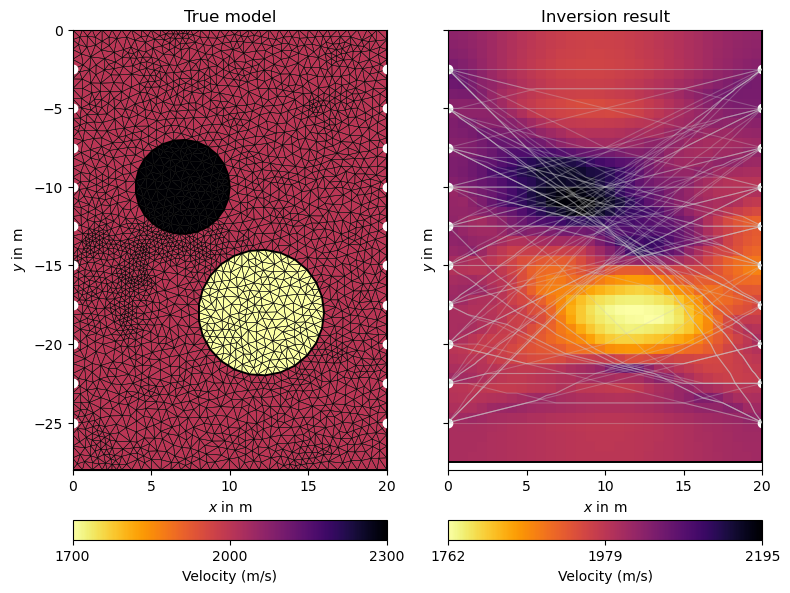# Crosshole traveltime tomography#

Seismic and ground penetrating radar (GPR) methods are frequently applied to image the shallow subsurface. While novel developments focus on inverting the full waveform, ray-based approximations are still widely used in practice and offer a computationally efficient alternative. Here, we demonstrate the modelling of traveltimes and their inversion for the underlying slowness distribution for a crosshole scenario.

We start by importing the necessary packages.

```import matplotlib.pyplot as plt
import numpy as np

import pygimli as pg
import pygimli.meshtools as mt
import pygimli.physics.traveltime as tt

pg.utils.units.quants['vel']['cMap'] = 'inferno_r'
```

## Geometry setup#

Next, we build the crosshole acquisition geometry with two shallow boreholes.

```# Acquisition parameters
bh_spacing = 20.0
bh_length = 25.0
sensor_spacing = 2.5

world = mt.createRectangle(start=[0, -(bh_length + 3)], end=[bh_spacing, 0.0],
marker=0)

depth = -np.arange(sensor_spacing, bh_length + sensor_spacing, sensor_spacing)

sensors = np.zeros((len(depth) * 2, 2))  # two boreholes
sensors[len(depth):, 0] = bh_spacing  # x
sensors[:, 1] = np.hstack([depth] * 2)  # y
```

Traveltime calculations work on unstructured meshes and structured grids. We demonstrate this here by simulating the synthetic data on an unstructured mesh and inverting it on a simple structured grid.

```# Create forward model and mesh
c0 = mt.createCircle(pos=(7.0, -10.0), radius=3, nSegments=25, marker=1)
c1 = mt.createCircle(pos=(12.0, -18.0), radius=4, nSegments=25, marker=2)
geom = world + c0 + c1
for sen in sensors:
geom.createNode(sen)

mesh_fwd = mt.createMesh(geom, quality=34, area=0.25)
model = np.array([2000., 2300, 1700])[mesh_fwd.cellMarkers()]
ax, cb = pg.show(mesh_fwd, model, logScale=False,
label=pg.unit('vel'), cMap=pg.cmap('vel'), nLevs=3)
```## Synthetic data generation#

Next, we create an empty DataContainer and fill it with sensor positions and all possible shot-receiver pairs for the two-borehole scenario.

```scheme = tt.createCrossholeData(sensors)
```

The forward simulation is performed with a few lines of code. We initialize an instance of the Refraction manager and call its simulate function with the mesh, the scheme and the slowness model (1 / velocity). We also add 0.1% relative and 10 microseconds of absolute noise.

Secondary nodes allow for more accurate forward simulations. Check out the paper by Giroux & Larouche (2013) to learn more about it.

```mgr = tt.TravelTimeManager()
data = tt.simulate(mesh=mesh_fwd, scheme=scheme, slowness=1./model,
secNodes=4, noiseLevel=0.001, noiseAbs=1e-5, seed=1337)

ax, cb = tt.showVA(data, usePos=False)
```## Inversion#

Now we create a structured grid as inversion mesh

```refinement = 0.25
x = np.arange(0, bh_spacing + refinement, sensor_spacing * refinement)
y = -np.arange(0.0, bh_length + 3, sensor_spacing * refinement)
mesh = pg.meshtools.createGrid(x, y)

ax, _ = pg.show(mesh, hold=True)
ax.plot(sensors[:, 0], sensors[:, 1], "ro")

invmodel = mgr.invert(data, mesh=mesh, secNodes=3, lam=1000, zWeight=1.0,
print("chi^2 = {:.2f}".format(mgr.inv.chi2()))  # Look at the data fit
``````fop: <pygimli.physics.traveltime.modelling.TravelTimeDijkstraModelling object at 0x17f1fe660>
Data transformation: <pygimli.core._pygimli_.RTrans object at 0x2addfcdb0>
Model transformation: <pygimli.core._pygimli_.RTransLog object at 0x17f1ff740>
min/max (data): 0.0096/0.02
min/max (error): 0.17%/0.2%
min/max (start model): 5.0e-04/5.0e-04
--------------------------------------------------------------------------------
inv.iter 0 ... chi² =  192.94
--------------------------------------------------------------------------------
inv.iter 1 ... chi² =   26.63 (dPhi = 84.04%) lam: 1000.0
--------------------------------------------------------------------------------
inv.iter 2 ... chi² =    4.39 (dPhi = 75.15%) lam: 1000.0
--------------------------------------------------------------------------------
inv.iter 3 ... chi² =    2.71 (dPhi = 31.76%) lam: 1000.0
--------------------------------------------------------------------------------
inv.iter 4 ... chi² =    1.80 (dPhi = 20.53%) lam: 1000.0
--------------------------------------------------------------------------------
inv.iter 5 ... chi² =    1.38 (dPhi = 10.93%) lam: 1000.0
--------------------------------------------------------------------------------
inv.iter 6 ... chi² =    1.19 (dPhi = 4.42%) lam: 1000.0
--------------------------------------------------------------------------------
inv.iter 7 ... chi² =    1.18 (dPhi = 0.39%) lam: 1000.0
################################################################################
#                Abort criterion reached: dPhi = 0.39 (< 2.0%)                 #
################################################################################
chi^2 = 1.18
```

Finally, we visualize the true model and the inversion result next to each other.

```fig, (ax1, ax2) = plt.subplots(1, 2, figsize=(8, 7), sharex=True, sharey=True)
ax1.set_title("True model")
ax2.set_title("Inversion result")

ax, cb = pg.show(mesh_fwd, model, ax=ax1, showMesh=True,
label=pg.unit('vel'), cMap=pg.cmap('vel'), nLevs=3)

for ax in (ax1, ax2):
ax.plot(sensors[:, 0], sensors[:, 1], "wo")

mgr.showResult(ax=ax2, logScale=False, nLevs=3)
mgr.drawRayPaths(ax=ax2, color="0.8", alpha=0.3)
fig.tight_layout()
```## Coverage and ray paths#

Note how the rays are attracted by the high velocity anomaly while circumventing the low-velocity region. This is also reflected in the coverage, which can be visualized as follows:

```fig, ax = plt.subplots()
mgr.showCoverage(ax=ax, cMap="Greens")
mgr.drawRayPaths(ax=ax, color="k", alpha=0.3)
p = ax.plot(sensors[:, 0], sensors[:, 1], "ko")
```White regions indicate the model null space, i.e. cells that are not traversed by any ray.

Total running time of the script: (0 minutes 14.805 seconds)

Gallery generated by Sphinx-Gallery